767

Physics Properties Of Matter Level: High School

Archimedes Principle

A rock weighs 140 N in air and has a volume of 0.00278 m^3 .

The acceleration of gravity is 9.8 m/s^2

What is its apparent weight when submerged in water ? Answer in units of N .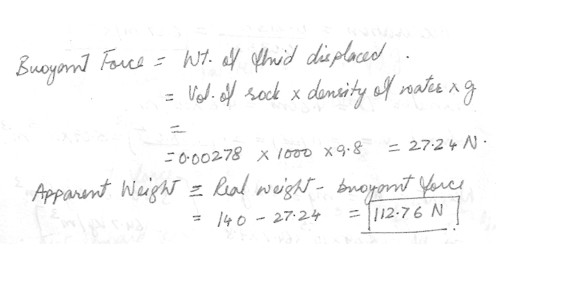766

Physics Properties Of Matter Level: High School

Pascal’s law

The small pistol of a hydraulic lift has a cross-sectional area of 8 cm^2 and the large piston has an area of 56 cm^2

What force F must be applied to the small piston to maintain the load of 58 kN at a constant elevation ? Answer in units of N .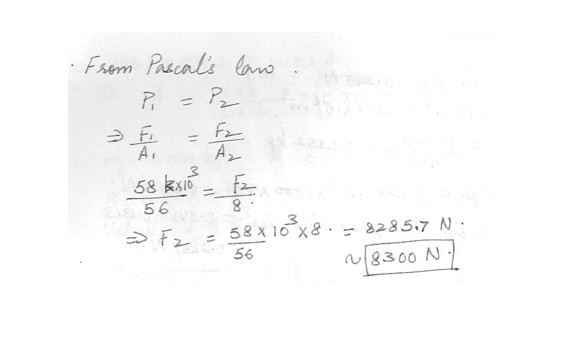765

Physics Properties Of Matter Level: High School

Pressure

What must be the contact area between a suction cup(completely exhausted ) and a ceiling in order to support the weight of an 32.7 kg student ?

The acceleration of gravity is 9.8 m/s^2 . Answer in units of m^2 .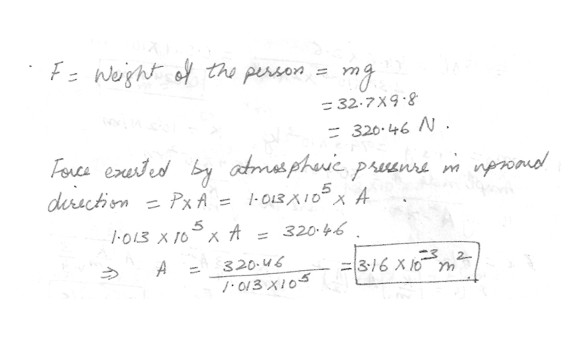760

Physics Properties Of Matter Level: High School

Solids

By how much does the wire stretch while the masses are in motion ? Answer in units of mm .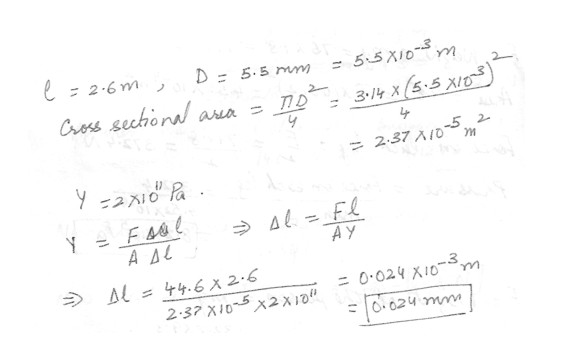759

Physics Properties Of Matter Level: High School

Solids

A 2.6 m long cylindrical steel wire with a cross-sectional diameter of 5.5 mm is placed over a frictionless pulley , with one end of the wire connected to a mass 6.5 kg and the other end to a mass 3.5 kg .

The acceleration of gravity is 9.8 m/s^2 . Given : Young’s modulus for steel Y = 2 x 10^11 Pa .

What is the tension in the wire while the masses are in motion ? Answer in units of N .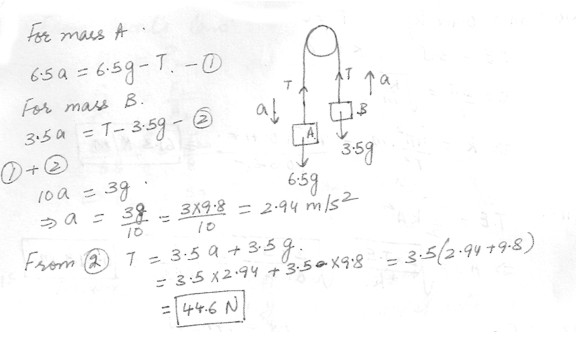757

Physics Properties Of Matter Level: High School

Solids

A stinless steel orthodontic wire is applied to a tooth that is out of line by 21 degree . The wire has an unstretched length of 4.5 cm and a diameter of 0.21 mm . If the wire is stretched 0.1 mm . find the magnitude of the force on the tooth . (Disregard to the width of the tooth). Young’s modulus for stainless steel is 1.8 x 10^11 Pa . Answer in units of N .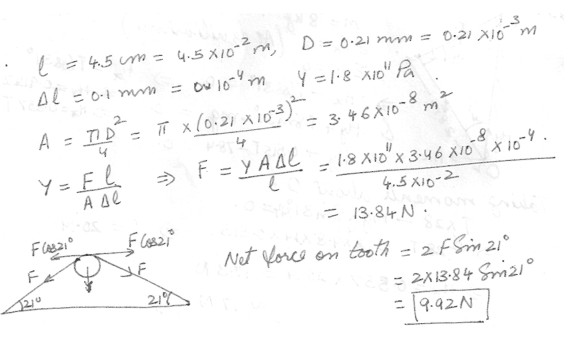756

Physics Properties Of Matter Level: High School

Solids

A 360 kg load is hung on a wire of length 4.5 m , cross sectional area 3.7 x 10^-5 m^2 , and Young’s modulus 8 z 10^10 N/m^2 .

The acceleration of gravity is 9.8 m/s^2 . What is its increase in length ? Answer in unit of mm .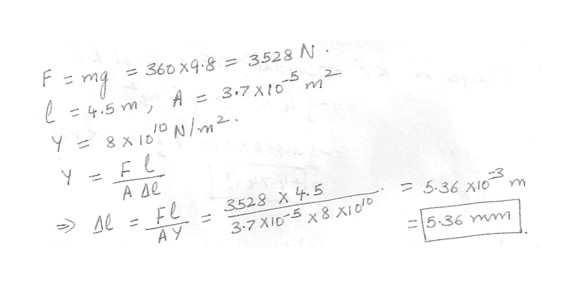755

Physics Properties Of Matter Level: High School

Solids

The elastic limit of copper is 1.82 x 10^8 N/m^2 . The acceleration of gravity is 9.8 m/s^2 . Determine the minimum diameter a copper wire can have under a load of 7.71 kg if its elastic limit is not be exceeded . Answer in units of m .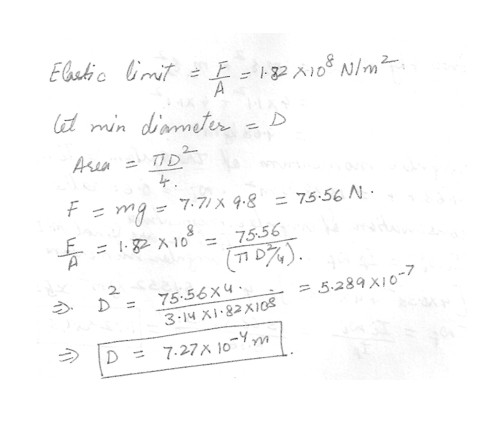751

Physics Properties Of Matter Level: High School

Explain the difference in molecular behavior for molecules in a solid compared each of molecules in a liquid and molecules in a gas . Assume that all three are the same temperature .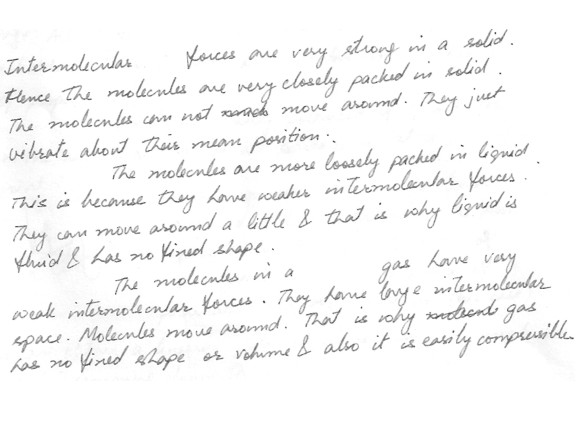707

Physics Properties Of Matter Level: High School

Aluminum has a positive coefficient of thermal expansion . Consider a round hole that has been drilled in a large sheet of Aluminum . As the temperature increases and the surrounding metal expands , the hole diameter will : Decrease , increase , depends how much metal surrounds the hole or remain constant ?
Increase

703

Physics Properties Of Matter Level: High School

Both the pressure and volume of a given sample of an ideal gas double . This means that its temperature in Kelvins must .

b. reduce to one-half its original value .

c. remain unchanged .

d. reduce to one-fourth its original value .

e. double .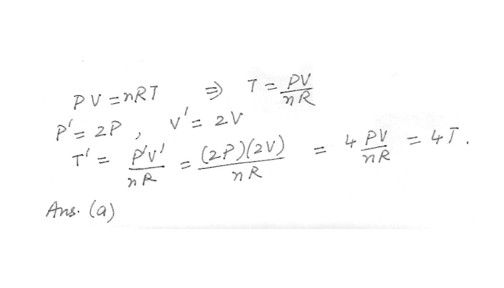702

Physics Properties Of Matter Level: High School

Properties of material

The weight that will cause a wire of diameter d to stretch a given distance, for a fixed length of wire, is

a. proportional to d.

b. proportional to d^2.

c. independent of what the length of the wire is.

d. independent of d.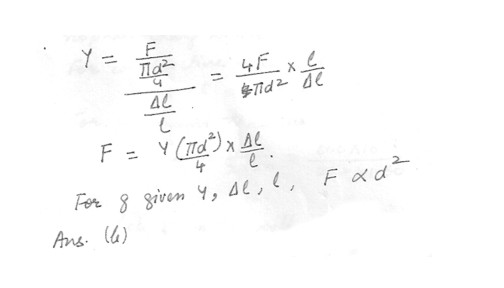Displaying 511-522 of 522 results.